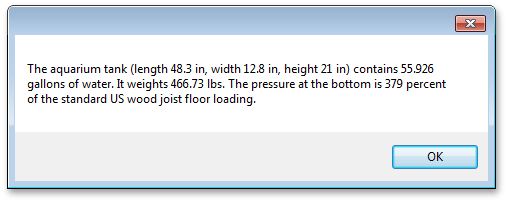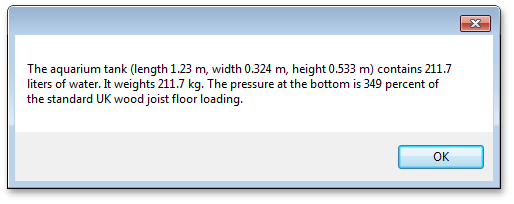.NET Framework 4.5.2+

# How to: Convert Between Metric and US Units of Measurement

This example illustrates the use of a QuantityValue<T> object and the Unit Conversion API converters for calculating the volume and the mass of the water in the aquarium tank; followed by a display of the results using US and metric units.

``````using DevExpress.UnitConversion;
//Quantity value is a value measured in a certain unit.
//You cannot mix different quantity values in a single expression.
//Instead, you can easily convert them as required and use the Value property to obtain numerical values.
QuantityValue<Distance> l = (48.25).Inches();
QuantityValue<Distance> w = (12.75).Inches();
QuantityValue<Distance> h = (21.0).Inches();
QuantityValue<Volume> vol = (l.ToMeters().Value * w.ToMeters().Value * h.ToMeters().Value).CubicMeters();
QuantityValue<Mass> m = (vol.ToLiters() * 1000).Value.Grams();
//US Standard uniform floor load is 0.2 psi.
//British standard imposed load is 1500 Newtons per square meter.

string textFormatUS = "The aquarium tank (length {0:g3} in, width {1:g3} in, height {2:g3} in) contains {3:g5} gallons of water. " +
"It weights {4:g5} lbs. " +
"The pressure at the bottom is {5:g3} percent of the standard US wood joist floor loading.";
string textUS = String.Format(textFormatUS, l.ToInches().Value, w.ToInches().Value,
h.ToInches().Value, vol.ToGallons().Value, m.ToPounds().Value, percentageUS);

string textFormatEu = "The aquarium tank (length {0:g3} m, width {1:g3} m, height {2:g3} m) contains {3:g5} liters of water. " +
"It weights {4:g5} kg. " +
"The pressure at the bottom is {5:g3} percent of the standard UK wood joist floor loading.";
string textEu = String.Format(textFormatEu, l.ToMeters().Value, w.ToMeters().Value,
h.ToMeters().Value, vol.ToLiters().Value, m.ToKilograms().Value, percentageEu);

string msg = radioButtonUS.Checked ? textUS : textEu;
MessageBox.Show(msg);
``````

For a US location, the output string looks like the following.For a UK location, the output string looks like the following.